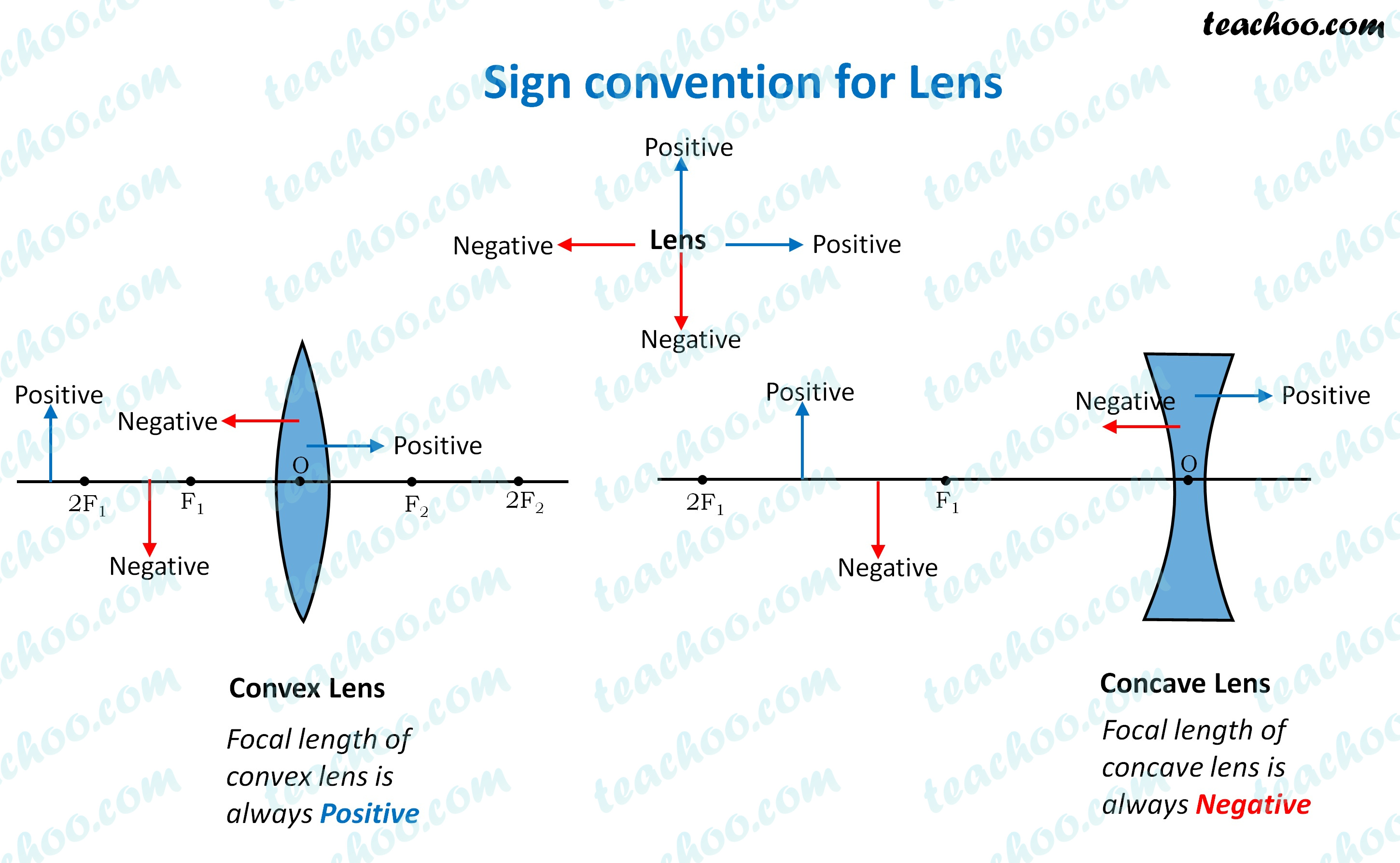MCQs from NCERT Exemplar

Class 10
Chapter 10 Class 10 - Light - Reflection and Refraction (Term 1)

## (d) A concave lens has –4 dioptre power having a focal length 0.25 m• A convex lens has
• a positive focal length ( 0.25m)
• and hence would also have a positive power (+4D).
• A concave lens has
• negative focal length (-0.25m)
• and hence has negative power ( -4D)

So, the correct answer is (a)